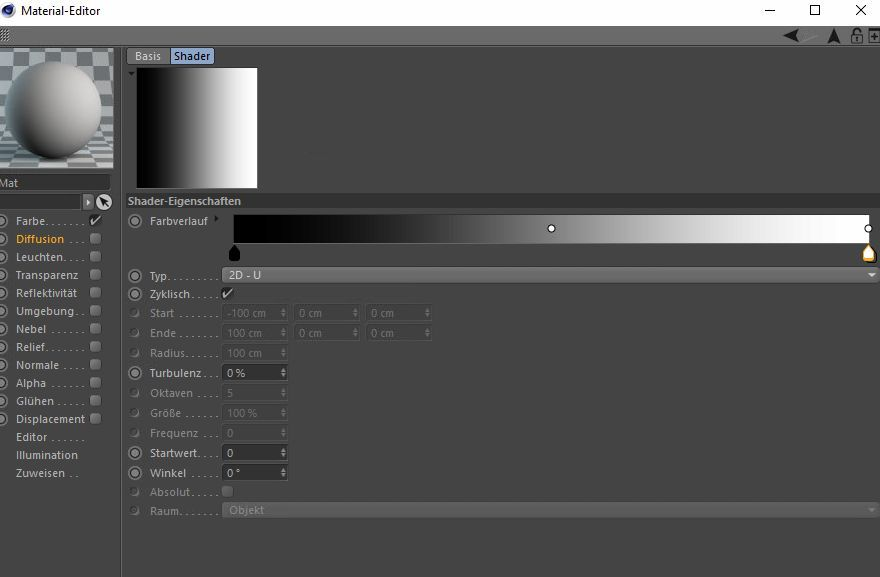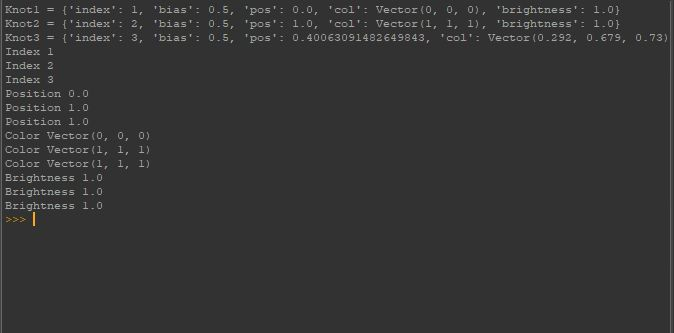# Solved Cinema Gradient, weird behaviour

Hi all,

I encountered a very weird behaviour. I tried to write a script in python which reads all the knot-values of one gradient and set those value to another gradient.
I can read the knots by calling GetKnot().
BUT when I try to extract a certain info from a Knot (for example the position) I get wrong results. So when transfering these infos to another gradient, the gradient is shown wrong.

Thats the Code:

``````import c4d
from c4d import gui

def main():
mat = doc.GetFirstMaterial()           #Gets the first material in the materials manager

mat.Message(c4d.MSG_UPDATE)
mat.Update(True, True)

print("Index" + " " + str(index1))
print("Index" + " " + str(index2))
print("Index" + " " + str(index3))
print("Position" + " " + str(pos1))
print("Position" + " " + str(pos2))
print("Position" + " " + str(pos3))
print("Color" + " " + str(col1))
print("Color" + " " + str(col2))
print("Color" + " " + str(col3))
print("Brightness" + " " + str(bri1))
print("Brightness" + " " + str(bri2))
print("Brightness" + " " + str(bri3))

mat.Message(c4d.MSG_UPDATE)
mat.Update(True, True)

# Execute main()
if __name__=='__main__':
main()
``````

And thats the result I get from the console and obviously the second gradient looks like that (wrong), too.

"Knot1 = {'index': 1, 'bias': 0.5, 'pos': 0.3, 'col': Vector(1, 1, 1), 'brightness': 1.0}
Knot2 = {'index': 2, 'bias': 0.5, 'pos': 1.0, 'col': Vector(1, 1, 1), 'brightness': 1.0}
Knot3 = {'index': 3, 'bias': 0.5, 'pos': 0.5, 'col': Vector(1, 0.6, 0.5), 'brightness': 1.0}
Index 1
Index 2
Index 3
Position 0.3
Position 1.0
Position 1.0
Color Vector(1, 1, 1)
Color Vector(1, 1, 1)
Color Vector(1, 1, 1)
Brightness 1.0
Brightness 1.0
Brightness 1.0
"

As you can see: When calling GetKnot() the infos of the Knots are right. But when extracting for example col of those Knots, The results are wrong. They all show Vector(1, 1, 1).

What is happening here?

Cheers,
PdZ

I coded a solution. But this was so much work and effort to get it work.
Its not very sexy though. BUT it works.

``````import c4d
from c4d import gui

def main():
mat = doc.GetFirstMaterial()           #Gets the first material in the materials manager

mat.Message(c4d.MSG_UPDATE)
mat.Update(True, True)

# index

# bias

# color

# position

# brightness

mat.Message(c4d.MSG_UPDATE)
mat.Update(True, True)

# Execute main()
if __name__=='__main__':
main()
``````

Hi, @PdZ in which version are you, using R20 with the first code works as expected, could you share a scene example?

Cheers,
Maxime.

MAXON SDK Specialist

@PdZ any news?

MAXON SDK Specialist

Sorry I was busy. Didn't meant to let you wait.

Iam working with:
Cinema 4D Studio R20 20.059

Heres the testscene:

Heres the code:

``````import c4d
from c4d import gui

def main():
mat = doc.GetFirstMaterial()           #Gets the first material in the materials manager

mat.Message(c4d.MSG_UPDATE)
mat.Update(True, True)

print("Index" + " " + str(index1))
print("Index" + " " + str(index2))
print("Index" + " " + str(index3))
print("Position" + " " + str(pos1))
print("Position" + " " + str(pos2))
print("Position" + " " + str(pos3))
print("Color" + " " + str(col1))
print("Color" + " " + str(col2))
print("Color" + " " + str(col3))
print("Brightness" + " " + str(bri1))
print("Brightness" + " " + str(bri2))
print("Brightness" + " " + str(bri3))

mat.Message(c4d.MSG_UPDATE)
mat.Update(True, True)

# Execute main()
if __name__=='__main__':
main()
``````There are 3 Knots. 2 at the same position on the right side.
Also the console tells me that result:PdZ

@PdZ

iam a stupid idiot. I had a typo.
Pls ignore everything. I checked the code and I set values of knot 2 to knot 3.

Iam sorry!

Don't feel sorry yes this is the issue and since Knot ID are not ordered from right to left but from creation order its why it's confused you.
Moreover, using a for loop will make way more sense and help you to avoid this kind of stuff.

``````    grad1 = shdr1[c4d.SLA_GRADIENT_GRADIENT]

``````

Finally, a BaseShader is derived from BaseList2D which is derived from C4DAtom that mean you can use GetClone to retrieves a clone of this shader so your code can look like.

``````import c4d

def main():
mat = doc.GetFirstMaterial()
return

mat.Message(c4d.MSG_UPDATE)
mat.Update(True, True)